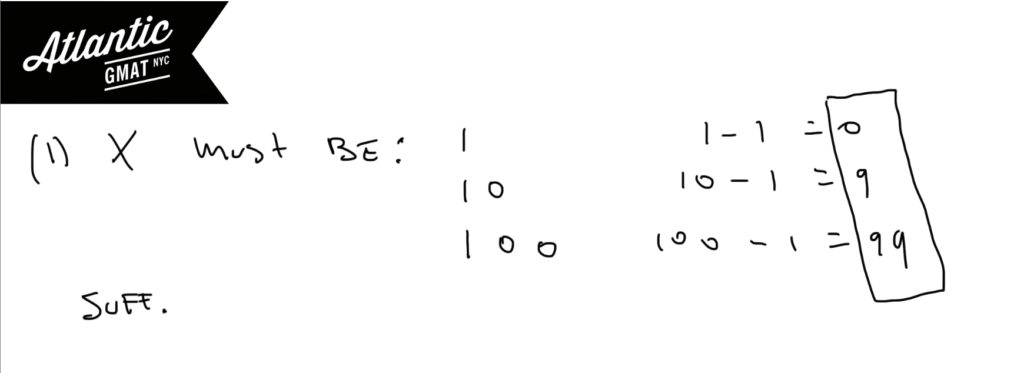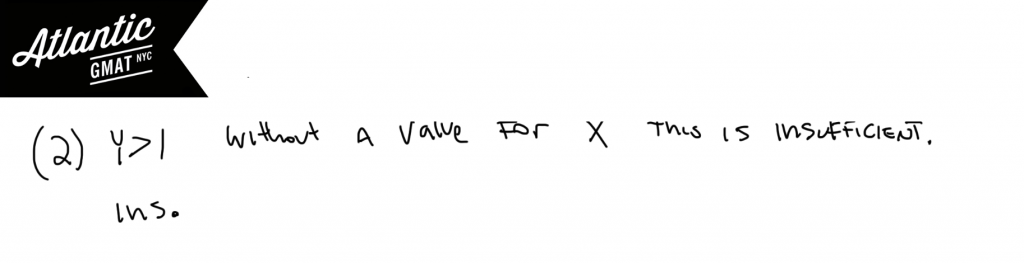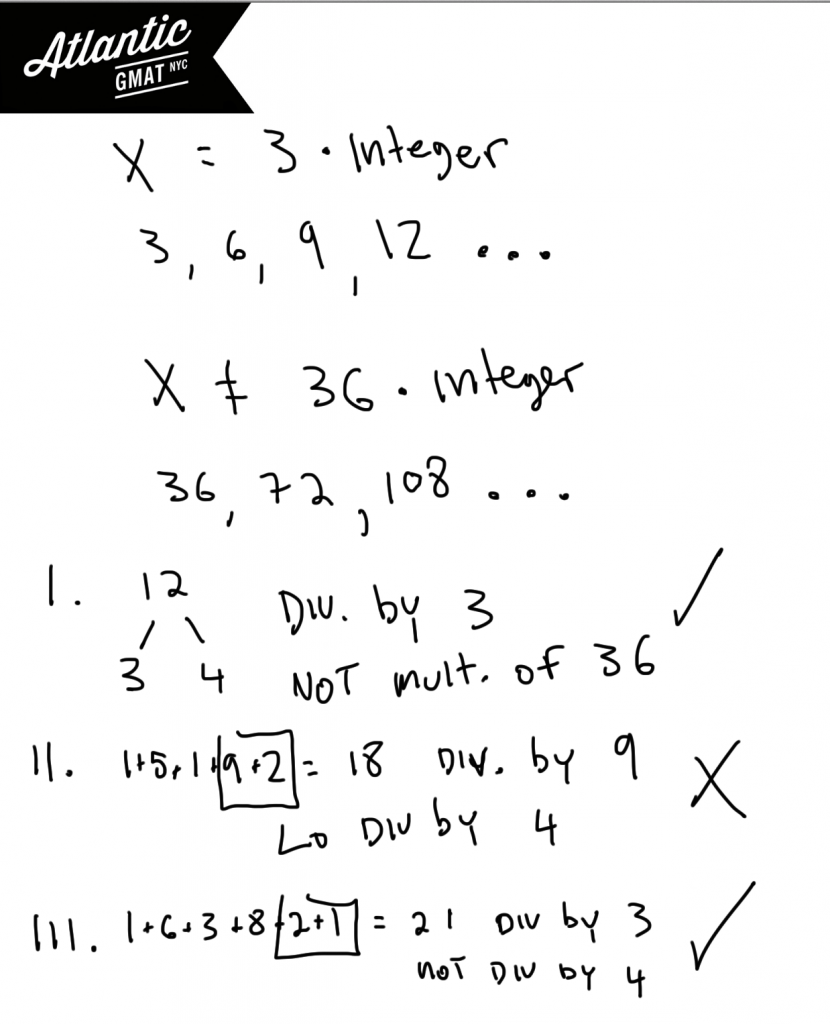Private GMAT Tutoring in NYC & Online

# GMAT Question of the Day - Data Sufficiency - Divisibility

What is the greatest common factor of positive integers k and m?

(1) When integer k is divided by two the result is an integer with no prime factors.

(2) m = 25

# GMAT Question of the Day - Data Sufficiency - Inequalities

In a certain forest 1/5 of the trees are Walnuts and 1/6 of the trees are Birches. What is the number of birch trees in the forest?

(1) The difference between the number of Walnut trees and the number of Birch trees is greater than 10

(2) There are fewer than 350 total trees

# GMAT Question of the Day - Data Sufficiency - Divisibility

If k is an integer is (k^2 - 7k + 12)(k+2)/4 an integer?

(1) k^2 - 1 is not divisible by 4

(2) k is divisible by 4

## GMAT Question of the Day Solution

For this GMAT question of the day you might consider that any set of 4 consecutive integers will have a one integer that is divisible by 4. 1, 2, 3, 4 or 7, 8, 9, 10. You also have to consider integer sets that have the same properties as a consecutive set: 1, 2, 3, 8 is the same as 1, 2, 3, 4 and 4, 9, 10, 11 is the same as 8, 9, 10, 11. So the integers don't have to be consecutive provided that you have 4 integers that have different remainders when divided by 4. If you have that then it is guaranteed that one of the integers will be divisible by 4.

Start this GMAT question of the day by factoring. Why? Because factoring will give you another way of looking at the numbers and in this case the factoring is simple. (k-3)(k-4)(k+2). So now we know that we have three numbers that have different remainders when divided by 4. How do we know that? None of the numbers are a multiple of 4 apart. That means that they are not in the same divisibility row. 4, 8, 12, 16, 20 are all in the same row of remainder 0. 1, 5, 9 are all remainder 1. 2, 6, 10 are all remainder 2. 3, 7, 11 are all remainder 3. We don't know if k-3 is remainder 0, 1, 2 or 3 but we do know that k-3 has a different remainder when divided by 4 than k-4 does. Same thing with k + 2. Now we are looking for the statements to help narrow down whether the missing one in the patter, in this case k-1, is divisible by 4. If we can figure that out then we can answer the question. If this concept is confusing re-read this and try to pick some real numbers to test all of this out.

Statement (1) is a difference of squares and should be factored: (k-1)(k+1) if this isn't divisibly by 4 then neither k-1 nor k+1 are divisible by 4. We already figured out above that if we know the divisibility of k -1 then we can answer the questions. But just to break it down farther: This means that k - 3 isn't divisible by 4 because it has the same divisibility by 4 as k +1. Therefore k-4 or k+2 (because k-1, k-2, k-3, k-4 are consecutive integers and k + 2 has the same divisibility by 4 as k - 2) must be divisible by 4 so the answer is yes. Sufficient.

Statement (2) If k is divisible by 4 then k - 4 must be divisible by 4 because they are in the same remainder row in terms of divisibility by 4. Pick some numbers to verify this. Sufficient.

# GMAT Question of the Day - Data Sufficiency - Number Properties/Divisibility

If x and y are both positive integers is (x^y - 22)/3 equal to an integer?

(1) The sum of the digits of x is equal to 1

(2) y > 1

## GMAT Question of the Day Solution

This question of the day deals with divisibility. Usually with this type of question it will help to make some kind of list or chart to see if there is some kind of pattern. Some GMAT tutoring students are resistant to making charts and lists on Data Sufficiency questions because this type of work hasn't paid off for them in the past. However, you have get a feeling for the types of questions that require making charts, lists, and writing out scenarios. There are plenty of times when these tools will make a DS question much simpler.

For this question you ought to think about the fact that every third number is divisible by 3. So 1, 2, 3 or 2, 3, 4, or 3, 4, 5. Those are all the different patterns for three. Notice that the number divisible by three can be in either of the three places as can the number that is 1 away from being divisible by 3 and the number that is 2 away from being divisible by 3. Notice that in terms of divisibility by 3, 1 is the same as 4; 2 is the same as 5. So there is a pattern. If we can figure out where x is in the pattern we'll be able to decide whether subtracting 1 will yield a number divisible by three.

(1) This tells us that x is either 1, 10, 100... That means that x will always be a number that is 1 away from divisible by 3. Therefore x-1 will always be divisible by three. You can also understand this with divisibility rules. If the sum of the digits equals a number that is divisible by three then the number itself is divisible by three. So if you know the sum of the digits of a number then you know what you can add/subtract to make that number divisible by 3. The same strategy will work for 9. Sufficient.(2) The value of y makes no difference provided that y is a positive integer. Larger values of y just add more zeroes after the one. That will not change the divisibility by 3. If y could have been negative then we would have had to consider its value because a negative y would have created a fraction. Insufficient.# GMAT Question of the Day - Problem Solving - Number Properties/Divisibility

If x is a positive multiple of 3 but not divisible by 36 then which of the following values could be a factor of x?

I. 12

II. 15192

III. 163821

A. I Only

B. II Only

C. III Only

D. I and III Only

E. I, II, and III

## GMAT Question of the Day Solution

I spend a lot time in GMAT tutoring sessions teaching how to recognize GMAT question types. By recognizing the question you can apply the right tools to get to the answer. When GMAT questions have such keywords as divisible by, multiple of, and factor of you should be thinking about divisibility and prime factors.  In this question of the day we have a few of these words and so should be considering the prime factors (or the very least the factors) of these numbers. We know that x is a positive multiple of 3 so that x could be 3, 6, 9... We also know that x is not a multiple of 36. So that x cannot have 9 and 4 as factors. In this case we don't need to worry about the complete prime factorization because it's easy enough to see what is happening. That means that x can be any multiple of 3 that is not divisible by both 9 and 4. Another way to say that is that x can't be a number with two 2's and 3's. x can have to two 2's but not two 3's or two 3's but not two 2's. We just can't have both of those numbers together because then the number will be divisible by 36 - a situation that is out of bounds in this question.

In the answer choices you need to eliminate answers that are either

1. Not divisible by 3

2. Divisible by both 4 and 9 (divisible by 36)

In this question and in many others you will be helped by knowing some basic divisibility rules. Any number whose digits add to 3 are divisibly by 3. Any number whose digits add to 9 is divisible by 9. If the last two digits of a number form a number that is divisible by 4 then the entire number is divisible by 4. You can use these rules to eliminate choice II. It is divisibly by both 9 and 4. Choices I and III both follow our rules.# CONTACT

Atlantic GMAT

405 East 51st St.

NY, NY 10022

(347) 669-3545

info@AtlanticGMAT.com